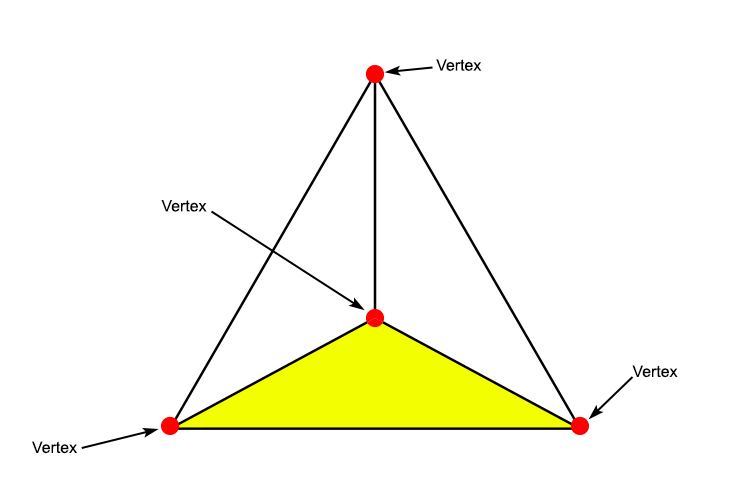Vertex

A vertex is where two or more line segments meet. A corner.

Plural of vertex is vertices (pronounced “ver-tiss-ease”).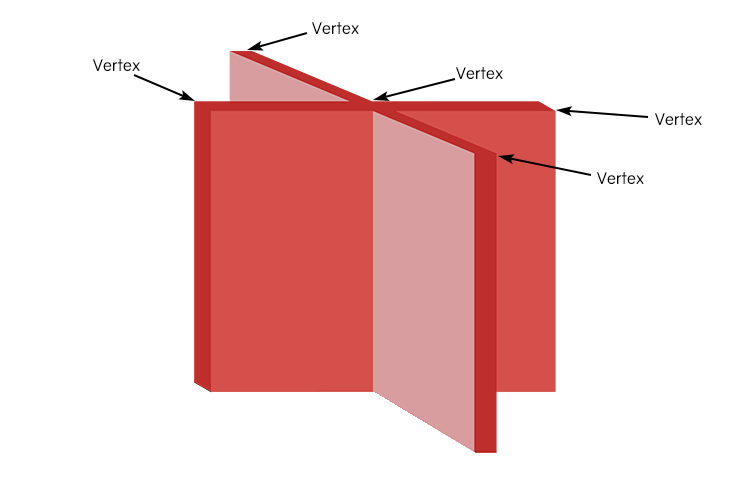A vertical X (vertex) is covered in points which have two lines meeting.

Example 1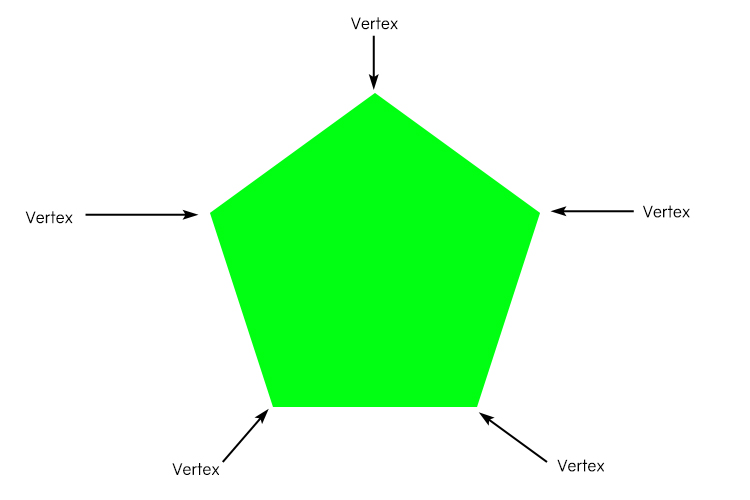A pentagon has five vertices

Example 2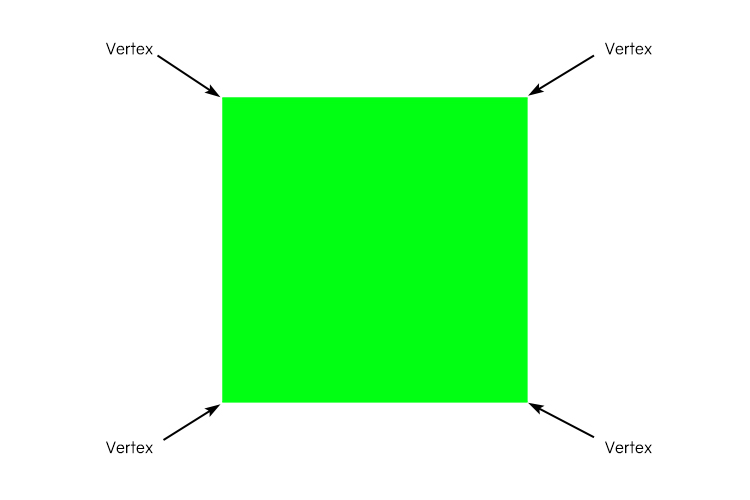A square has four corners but these are four vertices too.

Example 3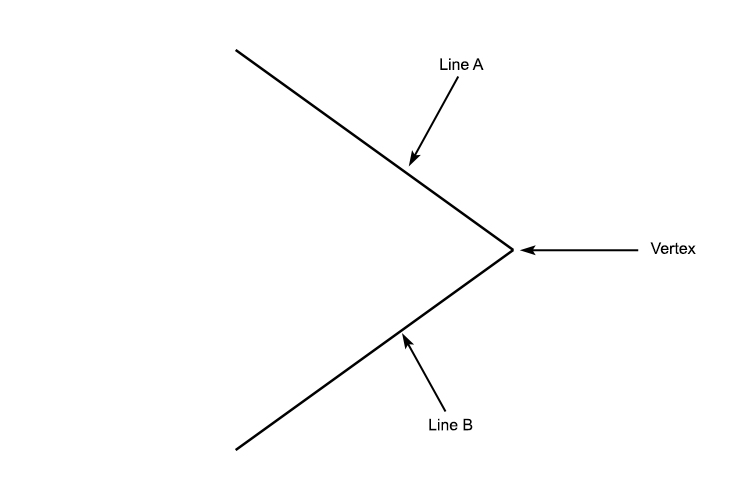Where two lines meet is a vertex.

Example 4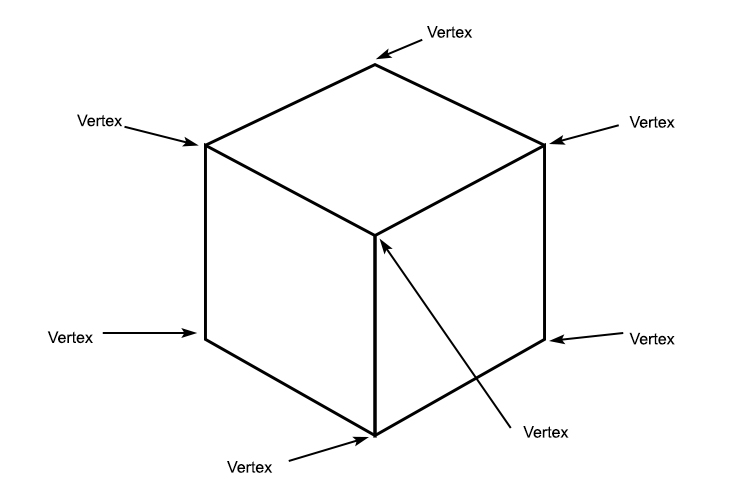Example 5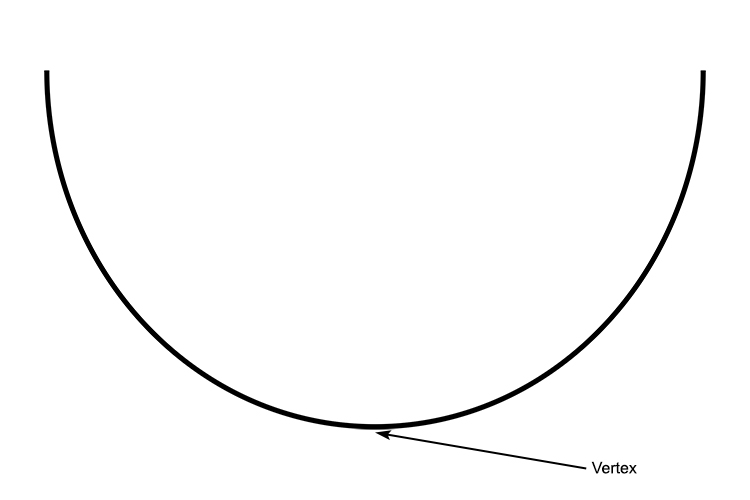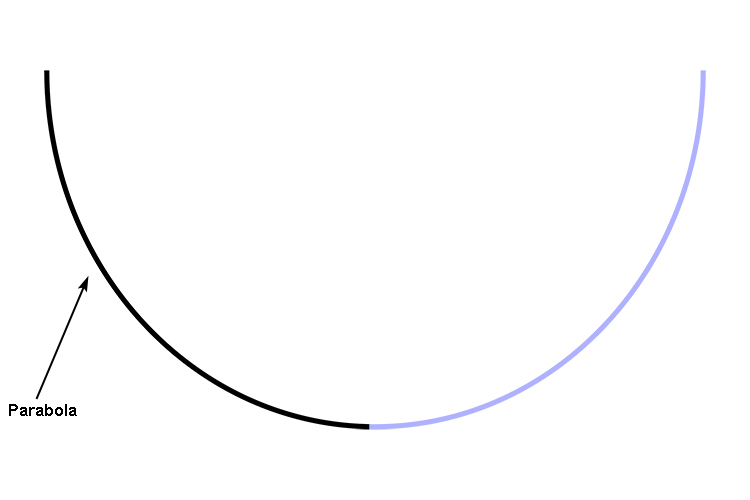A parabola can be thought of as two curves meeting, that is why mathematicians still call this a vertex. This can be the high point or low point of the graph.

Example 6